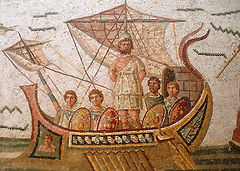Topics

All areas of pure and applied mathematics, such as (but not limited to):

Algebra

Analysis

Geometry

Topology

Fractional Calculus

Modeling and Numerical Analysis

Number Theory

Differential Equations

Stochastic Process and Their Applications

Probability and Statistics

Operations Research

Mathematical Finance

Mathematical Economics

Mathematical Physics

Bio-Mathematical

Bio-StatisticsINTERNATIONAL E-CONFERENCE ON PURE AND APPLIED

MATHEMATICAL SCIENCES (ICPAMS-2021)

7-10 June 2021